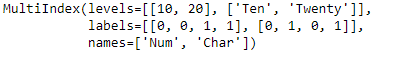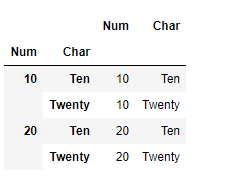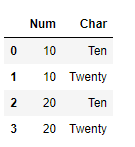Open in App
Not now

# Python | Pandas MultiIndex.to_frame()

• Last Updated : 24 Dec, 2018

Python is a great language for doing data analysis, primarily because of the fantastic ecosystem of data-centric python packages. Pandas is one of those packages and makes importing and analyzing data much easier.

Pandas` MultiIndex.to_frame()` function create a DataFrame with the levels of the MultiIndex as columns.

Syntax: MultiIndex.to_frame(index=True)

Parameters :
index : Set the index of the returned DataFrame as the original MultiIndex.

Returns : DataFrame : a DataFrame containing the original MultiIndex data.

Example #1: Use `MultiIndex.to_frame()` function to construct a dataframe using the MultiIndex levels as the column and index.

 `# importing pandas as pd``import` `pandas as pd`` ` `# Create the MultiIndex``midx ``=` `pd.MultiIndex.from_tuples([(``10``, ``'Ten'``), (``10``, ``'Twenty'``), ``                                  ``(``20``, ``'Ten'``), (``20``, ``'Twenty'``)], ``                                        ``names ``=``[``'Num'``, ``'Char'``])`` ` `# Print the MultiIndex``print``(midx)`

Output :Now let’s construct the dataframe from the MultiIndex.

 `# Construct the DataFrame``midx.to_frame(index ``=` `True``)`

Output :As we can see in the output, the function has constructed the Dataframe using the MultiIndex. Notice the index of the dataframe is constructed using the levels of the MultiIndex.

Example #2: Use `MultiIndex.to_frame()` function to construct a DataFrame using the MultiIndex. Do not use the MultiIndex levels to construct the index of the Dataframe.

 `# importing pandas as pd``import` `pandas as pd`` ` `# Create the MultiIndex``midx ``=` `pd.MultiIndex.from_tuples([(``10``, ``'Ten'``), (``10``, ``'Twenty'``),  ``                                  ``(``20``, ``'Ten'``), (``20``, ``'Twenty'``)], ``                                        ``names ``=``[``'Num'``, ``'Char'``])`` ` `# Print the MultiIndex``print``(midx)`

Output :Now let’s create a dataframe using the midx MultiIndex.

 `# Create Dataframe with new index values.``midx.to_frame(index ``=` `False``)`

Output :As we can see in the output, the function has returned a DataFrame having different index value.

My Personal Notes arrow_drop_up